### Home > CAAC > Chapter 4 > Lesson 4.1.4 > Problem4-34

4-34.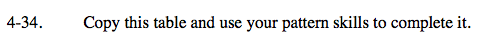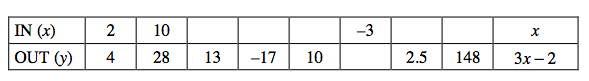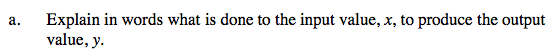See the table above.

The equation is y = 3x − 2.

Multiplty x by 3 and subtract 2.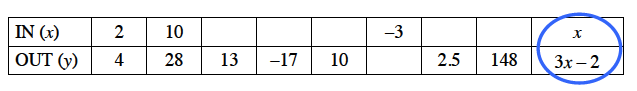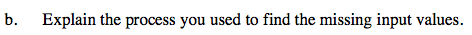How did you use the equation to solve for x?